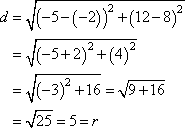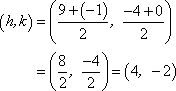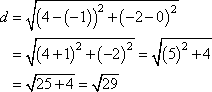Search

Conics: Circles: Working with Equations (page 2 of 3)

Sections: Introduction & Drawing, Working with equations, Further examples• State the radius and center of the circle with equation 16 = (x  2)2 + (y  3)2.
• The numerical side, the 16, is the square of the radius, so it actually indicates 16 = r2 = 42, so the radius is r = 4. Reading from the squared-variable parts, the center is at (h, k) = (2, 3).

• State the radius and center of the circle with equation 25 = x2 + (y + 3)2.
• The numerical side tells me that r2 = 25, so r = 5. The x-squared part is really (x  0)2, so h = 0. The temptation is to read off the "3" from the y-squared part and conclude that k is 3, but this is wrong. The center-vertex form has subtraction in it, so I need to convert first to that form.

y + 3 = y  (3)

So the y-coordinate of the center is actually k = 3.

radius r = 5, center (h, k) = (0, 3)

Warning: It is very easy to forget that sign in the middle of the squared parts. Don't be careless!

In the previous examples, information was extracted from a given equation. You'll also need to be able to work from given information backwards to find an equation.

• Find an equation for the circle with center (h, k) = (4, 2) and radius r = 10.
• I'll just plug the center and radius into the center-radius form:

(x  (4))2 + (y  (2))2 = 102
(x  4)2 + (y + 2)2 = 100

Since no particular form of the equation was specified, the above is an acceptable answer. If your book specifies some other format, then you may need to multiply things out:

x2  8x + 16 + y2 + 4y + 4 = 100
x2 + y2  8x + 4y + 20 = 100

x2 + y2  8x + 4y  80 = 0

Keep in mind that there is no standard meaning to the term "standard form". If your book specifies some other form, memorize that form for your tests.

• Find the center and radius of the circle with equation x2 + y2 + 2x + 8y + 8 = 0
• To convert to center-radius form, I'll need to complete the squares.

x2 + y2 + 2x + 8y + 8 = 0
x2 + 2x + [    ] + y2 + 8y + [    ] = 8 + [    ] + [    ]

x2 + 2x + [ 1 ] + y2 + 8y +  = 8 + [ 1 ] + 

(x + 1)2 + (y + 4)2 = 9

(x  (1))2 + (y  (4))2 = 32

Then the center is at (h, k) = (1, 4) and the radius is r = 3.

• Find an equation for the circle centered at (5, 12)
and passing through the point
(2, 8).
• Advertisement

The Distance Formula will give me the radius, which is, after all, the distance between the center and any point on the circle.Then the center-radius form of the equation is:

(x  (5))2 + (y  (12))2 = 52
(x + 5)2 + (y  12)2 = 25

• Find an equation for the circle with a diameter with endpoints at (9, 4) and (1, 0).
• The radius will be half the length of the diameter, and the midpoint of the diameter will be the center. The Midpoint Formula gives me:I can use either end of the diameter for my point on the circle; the distance between the center and the circle will be the same, regardless. I like smaller numbers, so I'll pick (1, 0). The Distance Formula gives me:This distance is the length of the radius r, so r2 = 29. Plugging my results into the center-radius form, I get: Copyright © Elizabeth Stapel 2010-2011 All Rights Reserved

(x  (4))2 + (y  (2))2 = 29
(x  4)2 + (y + 2)2 = 29

<< Previous  Top  |  1 | 2 | 3  |  Return to Index  Next >>

 Cite this article as: Stapel, Elizabeth. "Conics: Circles: Working with Equations." Purplemath. Available from     https://www.purplemath.com/modules/circle2.htm. Accessed [Date] [Month] 2016

Study Skills Survey

Tutoring from Purplemath
Find a local math tutor

 Copyright © 2021  Elizabeth Stapel   |   About   |   Terms of Use   |   Linking   |   Site Licensing Contact Us